Publicité

# Matrix presentation

1 Feb 2023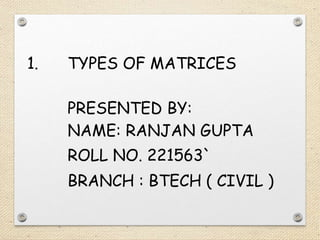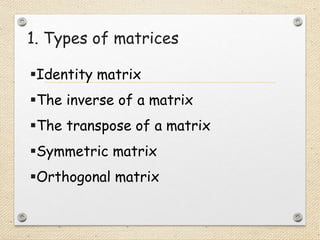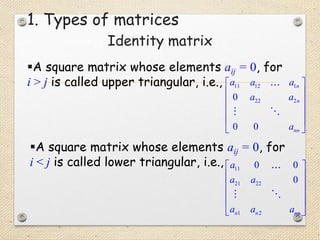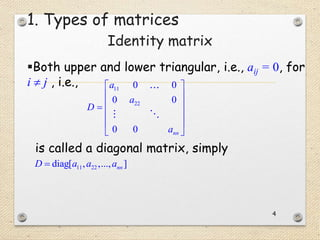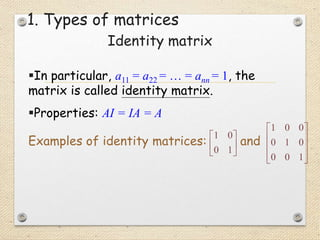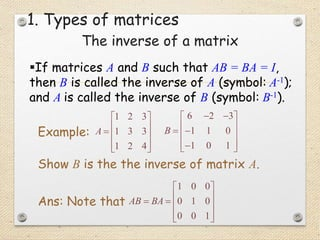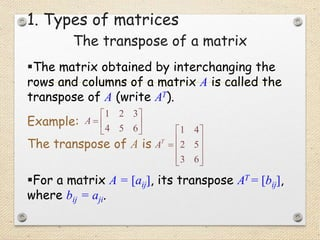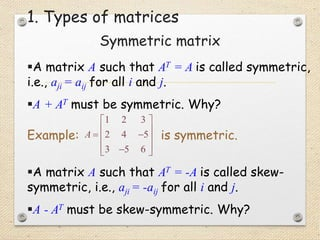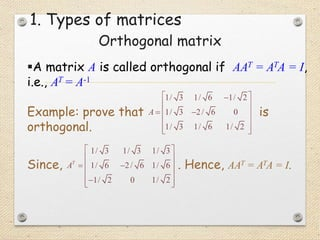1 sur 9
Publicité

### Matrix presentation

1. 1. TYPES OF MATRICES PRESENTED BY: NAME: RANJAN GUPTA ROLL NO. 221563` BRANCH : BTECH ( CIVIL )
2. 1. Types of matrices Identity matrix The inverse of a matrix The transpose of a matrix Symmetric matrix Orthogonal matrix
3. A square matrix whose elements aij = 0, for i > j is called upper triangular, i.e., 11 12 1 22 2 0 0 0             n n nn a a a a a a A square matrix whose elements aij = 0, for i < j is called lower triangular, i.e., 11 21 22 1 2 0 0 0             n n nn a a a a a a Identity matrix 1. Types of matrices
4. 4 Both upper and lower triangular, i.e., aij = 0, for i  j , i.e., 11 22 0 0 0 0 0 0              nn a a D a 11 22 diag[ , ,..., ]  nn D a a a Identity matrix 1. Types of matrices is called a diagonal matrix, simply
5. In particular, a11 = a22 = … = ann = 1, the matrix is called identity matrix. Properties: AI = IA = A Examples of identity matrices: and 1 0 0 1       1 0 0 0 1 0 0 0 1           Identity matrix 1. Types of matrices
6. If matrices A and B such that AB = BA = I, then B is called the inverse of A (symbol: A-1); and A is called the inverse of B (symbol: B-1). The inverse of a matrix 6 2 3 1 1 0 1 0 1 B                Show B is the the inverse of matrix A. 1 2 3 1 3 3 1 2 4 A            Example: 1 0 0 0 1 0 0 0 1 AB BA             Ans: Note that 1. Types of matrices
7. The transpose of a matrix The matrix obtained by interchanging the rows and columns of a matrix A is called the transpose of A (write AT). Example: The transpose of A is 1 2 3 4 5 6        A 1 4 2 5 3 6            T A For a matrix A = [aij], its transpose AT = [bij], where bij = aji. 1. Types of matrices
8. Symmetric matrix A matrix A such that AT = A is called symmetric, i.e., aji = aij for all i and j. A + AT must be symmetric. Why? Example: is symmetric. 1 2 3 2 4 5 3 5 6              A A matrix A such that AT = -A is called skew- symmetric, i.e., aji = -aij for all i and j. A - AT must be skew-symmetric. Why? 1. Types of matrices
9. Orthogonal matrix A matrix A is called orthogonal if AAT = ATA = I, i.e., AT = A-1 Example: prove that is orthogonal. 1/ 3 1/ 6 1/ 2 1/ 3 2/ 6 0 1/ 3 1/ 6 1/ 2                A 1. Types of matrices Since, . Hence, AAT = ATA = I. 1/ 3 1/ 3 1/ 3 1/ 6 2/ 6 1/ 6 1/ 2 0 1/ 2 T A               
Publicité